# 51

This post looks at some of the current models for how two agents can split the gain in non-zero sum interactions. For instance, if you and a buddy have to split £10 between the two of you, where the money is discarded if you can't reach a deal. Or there is an opportunity to trade your Elvis memorabilia for someone's collection of North Korean propaganda posters: unless you can agree to a way of splitting the gain from trade, the trade won't happen. Or there is the stereotypical battle of the sexes: either a romantic dinner (RD) or a night of Battlestar Galactica (BG) is on offer, and both members of the couple prefer doing something together to doing it separately - but, of course, each one has their preference on which activity to do together.

Unlike standard games such as Prisoner's Dilemma, this is a coordinated game: the two agents will negotiate a joint solution, which is presumed to be binding. This allows for such solutions as 50% (BG,BG) + 50% (RD,RD), which cannot happen with each agent choosing their moves independently. The two agents will be assumed to be expected utility maximisers. What would your feelings be on a good bargaining outcome in this situation?

Enough about your feelings; let's see what the experts are saying. In general, if A and C are outcomes with utilities (a,b) and (c,d), then another possible outcome is pA + (1-p)C (where you decide first, with odds p:1-p, whether to do outcome A or C), with utility p(a,b) + (1-p)(c,d). Hence if you plot every possible expected utilities in the plane for a given game, you get a convex set.

For instance, if there is an interaction with possible pure outcomes (-1,2), (2,10), (4,9), (5,7), (6,3), then the set of actual possible utilities is the pentagon presented here: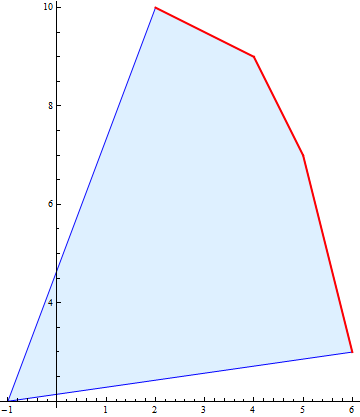The points highlighted in red are the Pareto-optimal points: those where there is no possibility of making both players better off. Generally only Pareto-optimal points are considered valid negotiation solutions: any other point leaves both player strictly poorer than they could be.

The first and instinctive way to choose an outcome is to select the egalitarian point. This is the Pareto-optimal point where both players get the same utility (in this instance, both get 27/5 utility):However, you can add an arbitrary number to your utility function without changing any of your decisions; your utility function is translation invariant. Since both players can do so, they can translate the shape seen above in any direction in the plane without changing their fundamental value system. Thus the egalitarian solution is not well-defined, but an artefact of how the utility functions are expressed. One can move it to any Pareto-optimal point simply with translations. Thus the naive egalitarian solution should be rejected.

Another naive possibility is to pick the point that reflects the highest total utility, by adding the two utilities together. This gives the vertex point (4,9):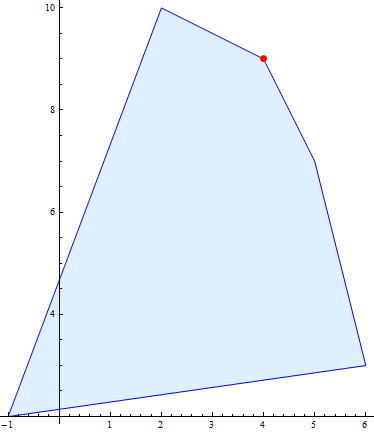This method will select the same outcome, no matter what translation is applied to the utilities. But this method is flawed as well: if one agent decides to multiply their utility function by any positive real factor, this does not change their underlying algorithm at all, but does change the "highest total utility". If the first agent decides to multiply their utility by five, for instance, we have the new picture portrayed here, and the "highest total utility point" has decidedly shifted, to the first player's great advantage: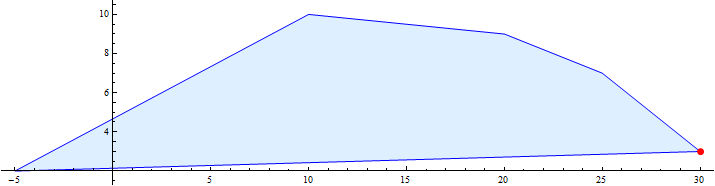To summarise these transformations to utility functions, we say that a utility function is defined only up to affine transformations - translations and scalings. Thus any valid method of bargaining must also be invariant to affine transformations of both the utility functions.

The two most popular ways of doing this are the Nash Bargaining solution (NBS), and the Kalai-Smorodinsky Bargaining Solution (KSBS). Both are dependent on the existence of a "disagreement point" d: a point representing the utilities that each player will get if negotiations break down. Establishing this disagreement point is another subject entirely; but once it is agreed upon, one translates it so that d=(0,0). This means that one has removed the translation freedom from the bargaining game, as any translation by either player will move d away from the origin. In this example, I'll arbitrarily choose the disagreement at d=(-1,4); hence it now suffices to find a scalings-invariant solution.

Nash did so by maximising the product of the (translated) utilities. There are hyperbolas in the plane defined by the sets of (x,y) such that xy=a; Nash's requirement is equivalent with picking the outcome that lies on the hyperbola furthest from the origin. The NBS and the hyperbolas can be seen here:This gives the NBS as (4,9). But what happens when we scale the utilities? This is equivalent to multiplying x and y by constant positive factors. This will change xy=a to xy=b, thus will map hyperbolas to hyperbolas. This also does not change the concept of "furthest hyperbola from the origin", so the NBS will not change. This is illustrated for the scalings x->(18/25)x and y->(19/18)y: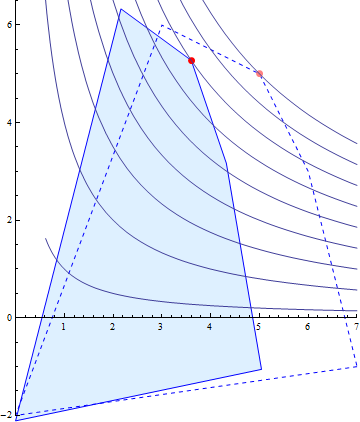The NBS has the allegedly desirable property that it is "Independent of Irrelevant Alternatives". This means that if we delete an option that is not the NBS, then the NBS does not change, as can be seen by removing (2,10):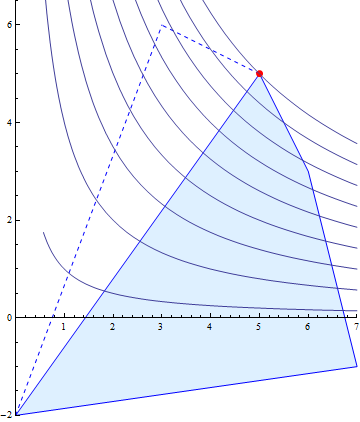Some felt that "Independence of Irrelevant Alternatives" was not a desirable property. In the above situation, player 2 gets their best option, while player 1 got the worse (Pareto-optimal) option available. Human intuition rebels against "bargaining" where one side gets everything, without having to give up anything. The NBS has other counter-intuitive properties, such as the that extra solutions can make some players worse off. Hence the KSBS replaces the independence requirement with "if one adds an extra option that is not better than either player's best option, this makes no player worse off".

The KSBS is often formulated in terms of "preserving the ratio of maximum gains", but there is another, more intuitive formulation. Define the utopia point u=(ux, uy), where ux is the maximal possible gain for player 1, and uy the maximal possible gain for player 2. The utopia point is what a game-theory fairy could offer the players so that each would get the maximal possible gain from the game. Then the KSBS is defined as the Pareto-optimal point on the line joining the disagreement point d with the utopia point u: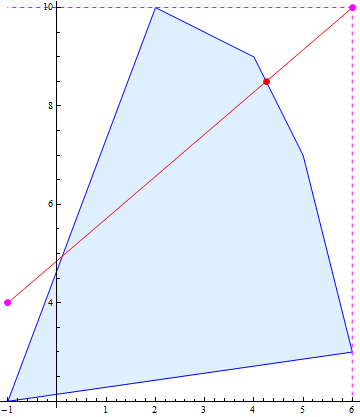Another way to formulate the KSBS is by renormalising the utilities so that d=(0,0), u=(1,1), and then choosing the egalitarian solution: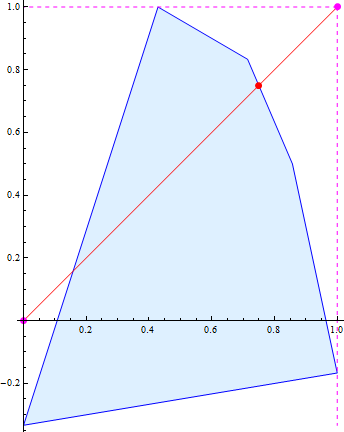In a subsequent post, I'll critique these bargaining solutions, and propose a more utility-maximising way of looking at the whole process.